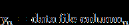## andBlock Category: Boolean

Inputs: Real, complex, or fixed-point scalars, or vectors or matrices.

Description: The and block generates the bitwise AND of 2 – 256 scalar input signals.

Right-click the and block to assign a different function to the block.

#### Example

1. Three variable and

Consider a variable y such that:

If a ≠ 6 and b > 2.2 and c < 7, then y = cos(t); else y = 0

where t is simulation time and is the input to all three parameters a, b, and c. This system is realized as:The output of the and block is true only when all the three inputs are true. This happens in the range t = (2.2, 7), except for the instant t = 6. This result is apparent in the upper plot. The variable y is equal to cos(t) in the range t = (2.2, 7). At the instant t = 6, variable a is momentarily false, and consequently, y = 0 at = 6 since the output of the and block evaluates to false at that instant.